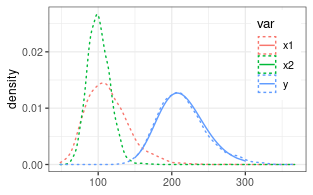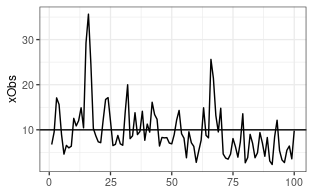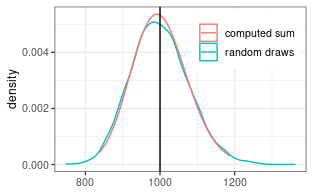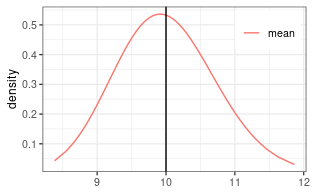# Approximating the sum of lognormal random variables

Lo 2013 derived the following formula for the approximation of the sum of several correlated lognormal random variables by a lognormal distribution. \begin{aligned} S_+ &= \operatorname{E}\left[\sum_i X_i \right] = \sum_i \operatorname{E}[X_i] = \sum_i e^{\mu_i + \sigma_i^2/2} \\ \sigma^2_{S} &= 1/S_+^2 \, \sum_{i,j} \operatorname{cor}_{ij} \sigma_i \sigma_j \operatorname{E}[X_i] \operatorname{E}[X_j] \\ &= 1/S_+^2 \, \sum_{i,j} \operatorname{cor}_{ij} \sigma_i \sigma_j e^{\mu_i + \sigma_i^2/2} e^{\mu_j + \sigma_j^2/2} \\ \mu_S &= \ln\left( S_+ \right) - \sigma_{S}^2/2 \end{aligned} where $$S_+$$ is the expected value of the sum, i.e the sum of the expected values of the terms. $$\mu_s$$ and $$\sigma_S$$ are lognormal distribution parameters of the sum, $$\mu_i$$ and $$\sigma_i$$ are the lognormal distribution parameters of the added random variables, and $$\operatorname{cor}_{ij}$$ is the correlation between two added random variables at log scale, which for time is computed from estimated autocorrelation $$\rho_k$$.

This method is implemented with function estimateSumLognormal, where the full correlation matrix is specified. For computational efficiency, the correlation length can be specified and correlations further apart will not contribute to the sum.

## Two uncorrelated random variables

# generate nSample values of two lognormal random variables
mu1 = log(110)
mu2 = log(100)
sigma1 = 0.25
sigma2 = 0.15
(coefSum <- estimateSumLognormal( c(mu1,mu2), c(sigma1,sigma2) ))
##        mu     sigma
## 5.3576474 0.1499077

A check by random numbers shows close correspondence.## Many correlated variables

### Generating observations and log-normally distributed random errors

We generate 10000 Observations of a sum of 100 random variables with mean 10 and multiplicative standard deviation of 1.7.

if (!requireNamespace("mvtnorm")) {
warning("Remainder of the vignette required mvtnorm installed.")
knitr::opts_chunk$set(error = TRUE) } nObs <- 100; nRep <- 10000 #nObs <- 1000; nRep <- 100 xTrue <- rep(10, nObs) sigmaStar <- rep(1.7, nObs) # multiplicative stddev theta <- getParmsLognormForExpval(xTrue, sigmaStar) # generate observations with correlated errors acf1 <- c(0.4,0.1) corrM <- setMatrixOffDiagonals( diag(nrow = nObs), value = acf1, isSymmetric = TRUE) xObsN <- exp(mvtnorm::rmvnorm( nRep, mean = theta[,1] , sigma = diag(theta[,2]) %*% corrM %*% diag(theta[,2]))) A single draw of the autocorrelated 100 variables looks like the following.### Estimating the correlation matrix and effective number of parameters We can estimate the autocorrelation matrix by assuming that it depends only on the distance in time, and estimate the autocorrelation matrix. The original autocorrelation function used to generate the sample was: ##  1.0 0.4 0.1 The effective autocorrelation function estimated from the sample is: (effAcf <- computeEffectiveAutoCorr(ds$xErr))
##  1.00000000 0.57197604 0.20089824 0.13886221 0.09787491
(nEff <- computeEffectiveNumObs(ds\$xErr))
##  33.51672

Due to autocorrelation, the effective number of parameters is less than nObs = 100.

### Computing the mean and its standard deviation

First we compute the distribution parameter of the sum of the 100 variables. The multiplicative uncertainty has decreased from 1.7.

#coefSum <- estimateSumLognormal( theta[,1], theta[,2], effAcf = effAcf )
coefSum <- estimateSumLognormal( theta[,1], theta[,2], effAcf = c(1,acf1) )
setNames(exp(coefSum["sigma"]), "sigmaStar")
## sigmaStar
##  1.077687

Its expected value corresponds to the sum of expected values (100*10).

(sumExp <- getLognormMoments( coefSum, coefSum)[1,"mean"])
## mean
## 1000

The lognormal approximation of the distribution of the sum, is close to the distribution of the 10000 repetitions.The mean is the sum divided by the number of observations, $$n$$. While the multiplicative standard deviation does not change by this operation, the location parameter is obtained by dividing by $$n$$ at original scale, hence, subtracting $$log(n)$$ at log-scale.

(coefMean <- setNames(c(coefSum["mu"] - log(nObs), coefSum["sigma"]), c("mu","sigma")))
##        mu     sigma
## 2.2997863 0.0748167

And we can plot the estimated distribution of the mean.